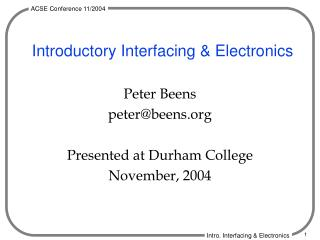DownloadDownload PresentationIntroductory Interfacing & Electronics

# Introductory Interfacing & Electronics

Télécharger la présentation## Introductory Interfacing & Electronics

- - - - - - - - - - - - - - - - - - - - - - - - - - - E N D - - - - - - - - - - - - - - - - - - - - - - - - - - -
##### Presentation Transcript

1. Introductory Interfacing & Electronics Peter Beens peter@beens.org Presented at Durham College November, 2004

2. Overview • This presentation covers a basic introduction to interfacing with the parallel port and just enough electronics to keep you from damaging your computer.

3. Interfacing Introduction

4. Interfacing Overview Computer Peripheral Interface • Wires • ICs • Resistors • Capacitors • Transistors • Connectors • LEDs • Motors • Lights • Robots • Joystick • Music Box

5. What Ports Can We Interface? • Parallel Port (AKA Printer Port) • Serial Port (AKA RS232) • Keyboard Port • USB??? (Hopefully soon…) We will concentrate on the Parallel Port

6. Identifying the Parallel Port • It’s the female connector with 25 pins • “DB25” Can be on a card

7. Electronics Introduction

8. Three Main Invisible Electrical Properties • Voltage, V, Volts • Provides the “push” • Current, I, Amperes (Amps) • Flow of Electrons • Amount of Current is dependent on Voltage and Resistance • Resistance, R, Ohms (S) • Limits the amount of current

9. Safe Current & Voltage Levels • Voltage: 30 V • Voltages inside a computer do not exceed 12 V, except at the power supply and power switch on older computers, which are at 120 V.Be careful in these areas! • Current: 5 mA (0.005 Amperes)

10. Voltage Sources

11. Current • Is the flow of electrons • Direction depends on convention

12. Ohm’s Law “Current (I) is proportional to Voltage (V) and inversely proportional to Resistance (R)”

13. Ohm’s Law & Power Wheel Reproduced by permission of Tony van Roon, 2002http://www.uoguelph.ca/~antoon

14. Kirchhoff’s Laws • Kirchhoff’s Voltage Law • “The sum of the voltage drops equals the applied voltage”, or… • “The sum of the voltage drops around a closed loop equals zero” • Used in series circuits • Kirchhoff’s Current Law • “The current entering a junction must equal the current leaving the junction” • Use in parallel circuits.

15. Light Emitting Diodes (LEDs) • A type of diode designed toemit light • Can be visible or IR • 2 V voltage drop • Typically draws 20 mA (0.020 A) • Schematic Symbol…

16. Resistors • Can be rated by… • Resistance (Ohms, S) • Tolerance (% of nominal value) • Power Rating (Watts) • Schematic Symbol…

17. Resistor Types

18. Resistor Colour Code Reproduced by permission of Tony van Roon, 2002http://www.uoguelph.ca/~antoon

19. Resistor Colour Code Example • 1st band: orange = 3 • 2nd band: orange = 3 • 3rd band: red = 2 (i.e. 102) • 4th band: gold = 5% 33 x 102 = 3300 S = 3.3 kS

20. Resistor Power Ratings

21. Series Circuits • One current path, therefore the current is the same everywhere • Total resistance is the sum of the individual resistances

22. Parallel Circuits • More than one current path • Total current is the sum of the individual currents

23. The Parallel Port – The Hardware

24. Parallel Port Specifications • Output Voltage • 0V for “low” • 5V for “high” • TTL (Transistor-Transistor Logic) • Output Current Limitation • 10-15 mA (careful!)

25. Parallel Port Pinout Graphic from http://www.doc.ic.ac.uk/~ih/doc/par/

26. Output Table

27. Input Table

28. Interfacing an LED Circuit

29. Understanding the LED Circuit • The parallel port output is 5V • A standard red LED needs ~20 mA and drops about 2 V • A resistor is needed to “drop” the excess voltage

30. Doing the Math From Kirchhoff’s Voltage Law From Ohm’s Law Currents equal in a series cct

31. 2N3904 TIP31 Motor Control (A stepper motor would require more outputs)

32. High Current Control • Use a relay

33. Parallel Port Connector Tip

34. The Programming

35. Turing: Preparing for Interfacing • Turing is already prepared for interfacing with the parallel port • No preparation necessary!

36. Turing: Turning On the LED • Parallelput(value) • Parallelput(1) turns on the 1 bit (D0) • Parallelput(255) turns on all bits (D0-D7)

37. Turing: Turning Off the LED • Parallelput(0)

38. Turing: Flashing the LED loop parallelput (1) delay (250) parallelput (0) delay (250) end loop

39. Turing: LED Walking loop % loops up for i : 0 .. 7 parallelput (2 ** i) delay (500) end for % loops down for decreasing i : 6 .. 1 parallelput (2 ** i) delay (500) end for end loop

40. Java: Preparing for Interfacing • Download http://www.geocities.com/Juanga69/parport/parport-win32.zip • Extract the parport folder to your classes folder • Copy parport.dll to you bin folder • import parport.ParallelPort; • ParallelPort lpt1 = new ParallelPort (0x378);

41. Java: Output ParallelPort lpt1 = new ParallelPort(0x378); int byteVal = 255; lpt1.write(byteVal); System.out.println("Output to port: " + byteVal);

42. Java: Input ParallelPort portIn = newParallelPort (0x378); int in; in = portIn.read (); System.out.println (in + " is currently being input.");

43. Java Delay Method private static void delay (int mS) { try{ Thread.sleep (mS); } catch (Exception e){ ; } }